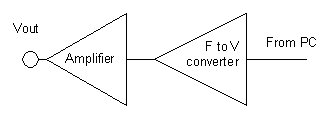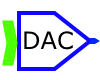# John Errington's Data Conversion Website

## Digital to Analog converters (DAC's)

There are several different ways in which a number from the computer can be converted to a corresponding value in the analog domain.  Most DACs take an input number and generate a current or voltage at the output.   The earliest (and easiest to understand) is the binary weighted resistor DAC. Resistive and current mode DACs are not much used now because other strategies (although harder to understand) are easier to implement on chip and provide faster more precise and accurate conversion.

#### Comment / application

binary weighted resistor not available 8 bits not manufactured now
R-2R ladder MAX 501 12 bits
current mode MAX 7624 8 bits
delta-sigma modulator CS4390 24 bits music
pulse width modulation     Motor speed control
frequency to voltage conversion ADVFC32.pdf    telemetry

### Characteristics of DACs

Resolution: This is the number of possible output levels the DAC is designed to reproduce. This is usually stated as the number of bits it uses, which is the base two logarithm of the number of levels. For instance a 1 bit DAC is designed to reproduce 2 (2^1) levels while an 8 bit DAC is designed for 256 (2^8) levels. Resolution is related to the effective number of bits (ENOB) which is a measurement of the actual resolution attained by the DAC.
Maximum sampling frequency: This is a measurement of the maximum speed at which the DACs circuitry can operate and still produce the correct output.
Monotonicity: This refers to the ability of a DAC's analog output to move only in the direction that the digital input moves (i.e., if the input increases, the output doesn't dip before asserting the correct output.) This characteristic is very important for DACs used as a low frequency signal source or as a digitally programmable trim element.
THD+N: This is a measurement of the distortion and noise introduced to the signal by the DAC. It is expressed as a percentage of the total power of unwanted harmonic distortion and noise that accompany the desired signal. This is a very important DAC characteristic for dynamic and small signal DAC applications.
Dynamic range: This is a measurement of the difference between the largest and smallest signals the DAC can reproduce expressed in decibels. This is usually related to DAC resolution and noise floor.

### Multiplying DAC's

A multiplying DAC gives a voltage out which is the product of the number it's converting and a reference voltage.  Depending on the design of the converter it may offer unipolar or bipolar operation. Unipolar operation means that the binary input can only be a positive number, whilst bipolar means that both positive and negative numbers can be used.  The converter may also support fixed, variable unipolar or variable bipolar reference voltages.

#### Here you can see the response of a unipolar DAC.  Some unipolar DAC's allow the reference voltage to be positive or negative.  This is called two quadrant operation.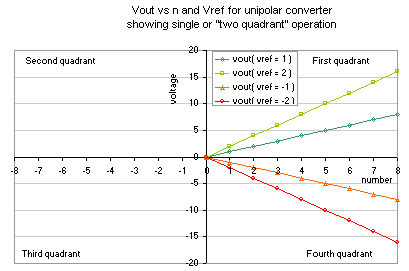#### In this figure you can see the response of a bipolar converter with a reference signal that can take either polarity.  This is called "four quadrant" operation.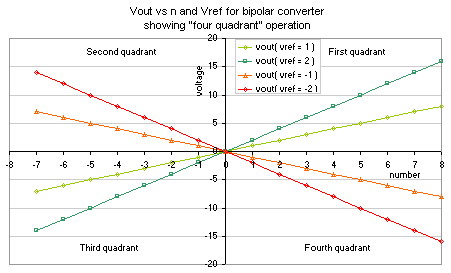### Binary Weighted Resisitor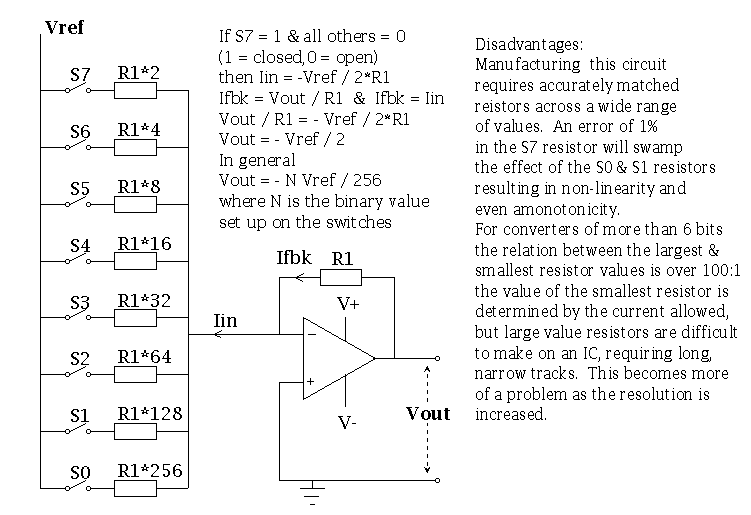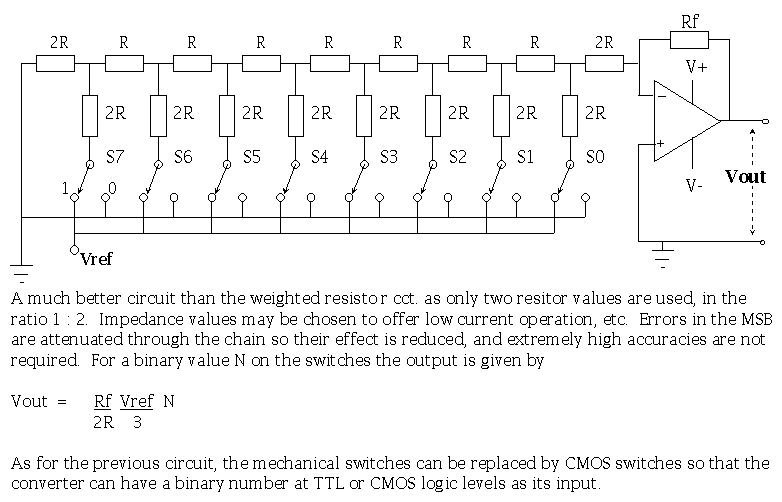### Voltage to Frequency conversion and Frequency to voltage conversion

These techniques are especially suited to use with digital equipment like microcomputers.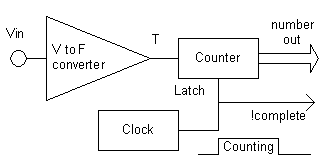#### Good V to F converters are capable of high conversion rates and high precision. Their accuracy can be limited and often subject to drift with temperature or age.